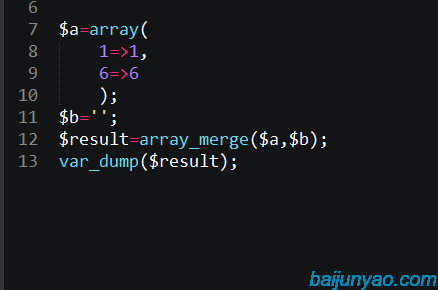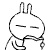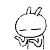# 深入解析array_merge函数的用法 php

array_merge是我们用来合并数组使用最多的函数；

1：如果数组中有相同的`字符串`键名；

``````\$a=array(
'a'=>'first a',
'b'=>'b'
);
\$b=array(
'c'=>'c',
'a'=>'second a'
);
\$result=array_merge(\$a,\$b);
var_dump(\$result);
\$result=\$a+\$b;
var_dump(\$result);``````

``````Array
(
[a] => second a
[b] => b
[c] => c
)``````

``````Array
(
[a] => first a
[b] => b
[c] => c
)``````

2：如果数组中有相同的`数字`键名、则格式化键名并保留全部的值；

``````\$a=array(
0=>'zero_a',
2=>'two_a',
3=>'three_a'
);
\$b=array(
1=>'one_b',
3=>'three_b',
4=>'four_b'
);
\$result=array_merge(\$a,\$b);
var_dump(\$result);``````

``````Array
(
 => zero_a
 => two_a
 => three_a
 => one_b
 => three_b
 => four_b
)``````

3：如果只传入一个数组；并且键名是数字；则格式化键名；

``````\$a=array(
1=>1,
3=>3,
6=>6
);
\$result=array_merge(\$a);
var_dump(\$result);``````

``````Array
(
 => 1
 => 3
 => 6
)``````

4：如果传的参数中有一个不是数组

``````\$a=array(
1=>1,
6=>6
);
\$b='';
\$result=array_merge(\$a,\$b);
var_dump(\$result);``````

``null``

``\$result=array_merge((array)\$a,(array)\$b);``• 总共14条评论2017-11-21 02:50:22 回复2017-11-27 13:57:31 回复2017-09-11 00:51:55 回复2017-06-06 06:03:55 回复2017-06-06 06:04:16 回复█.·〢習慣 ：我也遇到了，，加了好多判断，结果一样，看到这个恍然大悟

2017-04-18 02:55:11 回复2016-09-13 07:00:09 回复2016-08-24 09:15:12 回复我原来也遇到过这个坑。。。

2016-08-05 07:16:20 回复NULL2016-03-19 03:43:00 回复2016-01-20 00:59:42 回复NULL2016-03-19 03:43:18 回复NULL2016-03-19 03:43:27 回复NULL2016-03-19 03:43:34 回复